# CBSE Class 8 Maths Formulas

### Maths Formulas for Class 8

Many students consider mathematics as a scary subject and math formulas are often difficult to remember when you don’t understand them well. The negative approach towards any subjects will make a student reluctant to study that particular subject.Usually students feel jittery during the exam time and they fail to give their best shot. To make things easier and guide through different concepts in Maths, we will discuss the important CBSE Class 8 math formulas here.

##### https://www.ribblu.com/index.php/cbse/papers/class-8/mathematics/25

Algebraic  Expansions:

1. (a + b)2 = a2 + 2ab + b2
2. (a – b) = a2 – 2ab + b2
3. (a + b) (a – b) = a2 – b2
4. (x + a)(x + b) = x2 + (a + b)x + ab
5.  (x + a)(x – b) = x2 + (a – b)x – ab
6.  (x – a)(x + b) = x2 + (b – a)x – ab
7.  (x – a)(x – b)  = x2 – (a + b)x + ab
8.  (a + b)3 = a3 + b3 + 3ab(a + b)
9.  (a – b)3  = a3 – b3 – 3ab (a – b)
10. (x + y + z)2 = x2 + y2 + z2 + 2xy + 2yz + 2xz
11. (x + y – z)2  = x2 + y2 + z2 + 2xy – 2yz – 2xz
12. (x – y + z)2 = x2 + y2 + z2 – 2xy – 2yz + 2xz

13. (x – y – z)2  = x2 + y2 + z2 – 2xy + 2yz – 2xz

Temperature Conversions :

ºC  = 5959  ( ºF – 32 )  ºF = 9595  ( ºC + 32 )

Simple Interest  = P X T X R / 100

Pythagorean Theorem:

a+ b2  = c2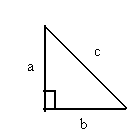Laws of Exponents
Multiplying powers with same base (Product Law)Dividing Powers with Same Base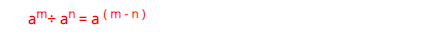Power of a Power ( Power law)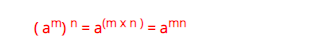Negative Powers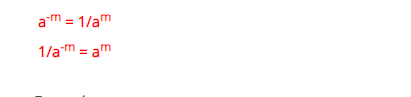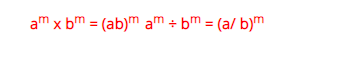Geometrical Formula: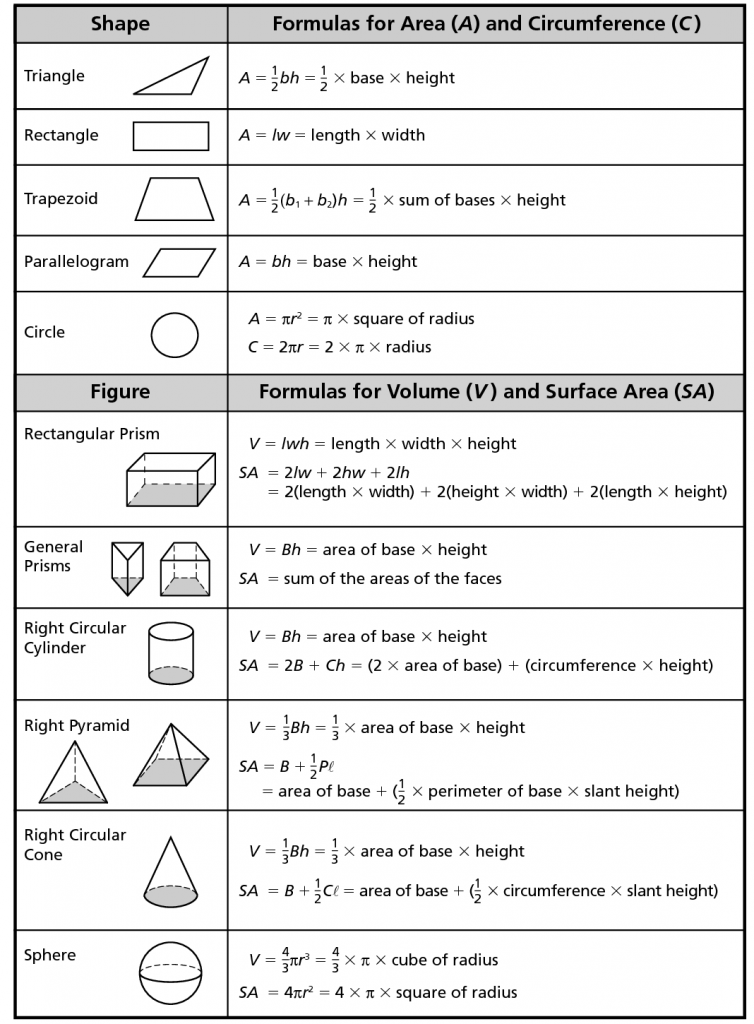Sl.No Figure Formula for surface area Formula for volume 1 Rectangular Prism SA = 2ab+2bc+2ca  sq. unitswhere a, b,c are the sides of the cube. V = abc  cubic units 2 Cylinder SA = 2πrh  sq. units TSA =  2πr(h+r)  sq. units r =radius of the cylinder h – height of the cylinder V = πr2h  cubic units 3 Cube SA = 6a2  sq. units a = sides of the cube V = a3    cubic units 4 Sphere SA = 4πr2  sq. units r = radius of the sphere V = 4343πr3   cubic units 5 Ellipsoid SA = 4π[(apbp+apcp+bpcp)3]1p4π[(apbp+apcp+bpcp)3]1pp = 1.6075a, b, c are semi axis of ellipsoid V = 4343 π r1,r2,r3  cubic units 6 Cone CSA = πr1 sq.units V = 1313πr2h   cubic units 7 Pyramid SA = a+1212*p*l p = perimeter of pyramid l = slant height a = area of the base of the pyramid V = 1313*a*h  cubic units 8 Torus SA =  π2 * (R2 – r2) R:Outer Radius r: Inner Radius V = 1414π3 (r1+r2) (r1-r2)2  cubic units 9 Hemisphere CSA = 2πr2 TSA = 3πr2  r = radius V = 2323 πr3   cubic units 10 Triangle SA = s(s−a)(s−b)(s−c)‾‾‾‾‾‾‾‾‾‾‾‾‾‾‾‾‾‾‾‾√s(s−a)(s−b)(s−c) where s is the perimeter of the triangle a, b, c are the sides of the triangle 11 Rectangle A = l*w L = length w = width 12 Triangle A = 1212bh b = base  h = height 13 Trapezoid A = 1212 h (b1+b2) 14 Parallelogram A = bh 15 Circle A = πr2   r = radius
/*]]>*/